# Acoustic impedance, intensity and power

 Acoustic impedance is the ratio of acoustic pressure to flow. It allows us to relate acoustic power and intensity to acoustic pressure and flow, and to calculate and the reflection and transmission at boundaries. This is a background page to the multimedia chapter Quantifying Sound. It uses some results from The wave equation for sound.

Acoustic impedance, which has the symbol Z, is the ratio of acoustic pressure p to acoustic volume flow U. (There is a close analogy with electrical impedance, the ratio of AC voltage V to current I.) The specific acoustic impedance is a ratio of acoustic pressure to specific flow, which is the same as flow per unit area, or acoustic flow velocity, u. So we define acoustic impedance Z and specific acoustic impedance z thus:

Z  =  p/U      and      z  =  p/u
In all cases, 'acoustic' refers to the oscillating component. With this proviso, we can say that
acoustic impedance  =  pressure/flow    and    specific acoustic impedance  =  pressure/velocity

The specific acoustic impedance, z, is an intensive property of a medium. We can specify the z of air or of water. The acoustic impedance Z is the property of a particular geometry and medium: we can discuss for example the Z of a particular duct filled with air. Usually, Z varies strongly when the frequency changes. The acoustic impedance at a particular frequency indicates how much sound pressure is generated by a given acoustic flow at that frequency.

Now let's get quantitative. We'll need the equation for a travelling sound wave that we found when we set up and solved the sound wave equation. For a one dimensional (plane) wave travelling to the right, these are shown in the animation below.

On the page on the sound wave equation, we wrote a solution for the particle displacement y as a function of position x and time t:

y  =  A sin (kx − ωt)

where A is the displacement amplitude, k is the wave number (k  =  2π/λ) and ω is the angular frequency (ω  =  2πf). We also showed that the particle velocity u and the acoustic pressure p are given by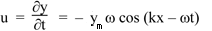and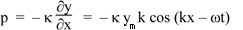where κ is the adiabatic bulk modulus. We defined z as p/u, so

z  =  κk/ω.

We also showed on that page that κ/ρ  =  v2, above so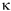=  ρv2 where ρ is the density. We also had ω/k  =  v. Substituting these gives

z  =  ρv.

Values for air and water. For air, the density is 1.2 kg.m−3 and v is 343 m.s−1, so the specific acoustic impedance for air is 420 kg.s−1.m−2  =  420 Pa.s.m−1. The values for condensed phases are usually much higher than those of gases. For (fresh) water, the density is 1000  kg.m−3 and v is 1480  m.s−1, so the specific acoustic impedance for water is 1.48 MPa.s.m−1. For human soft tissues, the values are comparable with that of water.

### Volume flow and acoustic impedance

Let’s now consider a one dimensional wave passing through an aperture with area A. The volume flow U is the volume passing per second through the aperture. If the flow moves a distance dy = udt, then the volume passing through is Ady, soThe acoustic impedance Z is the ratio of sound pressure to volume flow, so provided that the wave is only one dimensional, we should have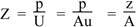In practice, it’s usually more complicated: if you send a wave into a pipe with uniform cross section A, there are usually reflections from the other end of the pipe, whether open or closed. So there is a sum of waves travelling to the right and left*. In the absence of reflections, we call this the characteristic acoustic impedance Z0. So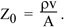It is possible to have no reflections, however. If the pipe is very long, then it takes a long time for the reflection to return and, when it does, it is much attenuated by losses at the wall. I mention this because, in our research laboratory, we introduced acoustically infinite acoustic waveguides as calibrations for acoustic measurements. The technique allows precise measurements of impedance spectra over 9 octaves using a single impedance head. It is described in this scientific paper.

* The reflections and resultant standing waves are very important in musical instruments. On the page What is acoustic impedance?, we give examples of acoustic impedance spectra for pipes and for musical instruments, and explain the importance of the quantity in music acoustics.

### Intensity and power as functions of time

The equations and animations above give the pressure and velocity. These are depicted in the animation below. Consider flow through an area A whose normal is in the direction that the wave is travelling. Consider the force F that is exerted on that plane by the oscillating air. From the definition of pressure,  F  =  pA.   The velocity of that moving air is u. The power exerted by a force applied at a point moving with speed v in the same direction is Fu. So the instantaneous power PInst transmitted is
PInst  =  Fu  =  pAu
where the subscript reminds us that PInst, F, u and p are all quantities that are varying with time, as shown in this animation. Note that, for a one dimensional wavel, p and u are in phase so, when they are both positive, PInst is positive and, when they are both negative, PInst is still positive.

We previously defined the intensity as the power transmitted per unit area. (See Travelling Waves II to revise.) So the instantaneous intensity due to a sound wave is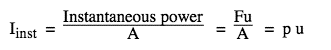Above we defined  z  =  p/u. We may rearrange this and use the result above that  z  =  ρv  to obtainwhich we substitute in the previous equation to give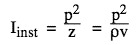For the sinusoidal oscillation we are considering, let the pressure amplitude be pm, so### Average intensity and RMS values

So the instantaneous power is proportional to the square of the pressure, as shown in this gave. We are usually interested in the average value of I, for which we need the mean value of p2(t).We've seen in Travelling Waves II that the average of a sine squared or cos squared function, over a whole number of cycles, is ½.The magnitude of a sound wave is sometimes described in terms of the pressure amplitude pm. Much more common, however, is to specify the RMS pressure: this is the square root of the mean of the square of the pressure. (See Power and RMS for more details.) Because the average value of p2(t) is ½,which we can represent graphicallyIf you see the acoustc pressure of a sound wave written as p, with no indication that it is the instantaneous value, it will nearly always mean the RMS value. So that allows us to drop the ½ in the previous expression and write the result for the average intensity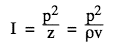Note that this equation is also true for instantaneous values. We can also put it in terms of the rms particle velocity u and displacement y:

I  =  zu2  =  ρcu2  =  ρcω2y2

and we can put it in terms of the maximum particle velocity um and displacement ym:

I  = ½ zum2  =  ½ ρcum2  = ½  ρcω2ym2

### Applications and implications

As we noted above, z varies greatly among media, especially between gas and condensed phases. Water is 800 times denser than air and its speed of sound is 4.3 times greater than that of air. So the specific acoustic impedance of water is 3500 times higher than that of air. This means that a sound in water with a given pressure amplitude is 3500 times less intense than one in air with the same pressure. This is because the air, with its lower z, moves with a much greater velocity and displacement amplitude than does water. Reciprocally, if a sound in water and another in air have the same intensity, then the pressure is much smaller in air.

Remember that the specific acoustic impedance, z, is an intensive property of a medium while acoustic impedance Z is the property of a particular geometry and medium: we can discuss for example the Z of a particular duct.

As mentioned above Z usually depends strongly on frequency. A few simple cases are worth examining in detail. When a small volume is compressed by a sound wave, the volume flow in acts to compress the air and raise its pressure. So it acts as a compliance. When a small volume is is accelerated by the difference in sound pressure, acoustic flow is determined by pressure difference and we have an acoustic inertance. We introduce these in Compliance, Inertance and Impedance.

On the site What is acoustic impedance?, we give examples of acoustic impedance spectra for pipes and for musical instruments, and explain the importance of the quantity in music acoustics. See this web site for the research lab and this scientific paper for some technical information. See also Acoustic impedance measurement techniques.This work is licensed under a Creative Commons License.